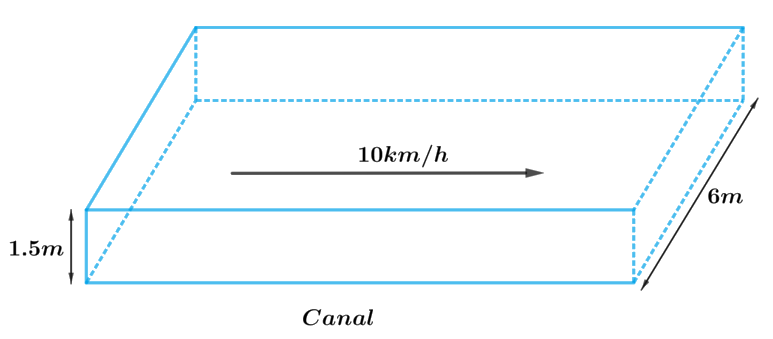# Ex.13.3 Q8 Surface Areas and Volumes Solution - NCERT Maths Class 10

Go back to  'Ex.13.3'

## Question

Water in canal, $$6\,\rm{m}$$ wide and $$1.5\,\rm{m}$$ deep, is flowing with a speed of $$10\,\rm{km/h}$$. how much area will it irrigate in $$30$$ minutes, if $$8\,\rm{cm}$$ of standing water is needed?

## Text Solution

What is known?

Width of the canal is $$6\rm{m}$$ and depth is $$1.5\rm{m}$$ and speed of flowing water is $$10\rm{km/h}$$

Height of the standing water is $$8\rm{cm}$$ in the area to be irrigated for $$30$$ minutes.

What is unknown?

The area to be irrigated in $$30$$ minutes with $$8\rm{cm}$$ of standing water.

Reasoning:

Draw a figure to visualize the shapes betterFrom the figure it’s clear that shape of the cross-section of the canal is cuboidal. So, volume of the water, flowing at the speed of $$10\rm{km/h}$$ for $$30$$ minutes will be same as the volume of water to irrigate the area with $$8\rm{cm}$$ of standing water.

To find the volume of the water we need to find length of the water flowing through canal in $$30$$ minutes at the speed of $$10\rm{km/h}$$

Hence, length of the water flowing through canal in $$1$$ hour $$= 10\rm{km}$$

Length of the water flowing through canal in $$30$$ minutes $$= 5\rm{km} = 5000\rm{m}$$

We will find the volume of the water by using formula;

Volume of the cuboid $$= lbh$$

where $$l, b$$ and $$h$$ are length, breadth and height of the cuboid respectively.

Volume of the water to irrigate the area $$=$$ Area to be irrigated $$\times$$ height of the standing water

Volume of water flowing through the canal in $$30$$ minutes $$=$$ Area to be irrigated $$\times$$ height of the standing water

Width of the cuboidal canal, $$b = 6m$$

Depth of the cuboidal canal,$$h = 1.5m$$

Speed of water flowing through the canal is $$10\rm{km/h}$$

Length of the water flowing through canal in $$1$$ hour $$(60\rm{minutes)}= 10\rm{km}$$ Length of the water flowing through canal in $$30$$ minutes\begin{align} = \frac{{30}}{{60}} \times 10km = 5km\end{align}

$l = 5 \times 1000m = 5000m$

Height of the standing water,\begin{align}{h_1} = 8cm = \frac{8}{{100}}m = 0.08m\end{align}

Volume of water flowing through the canal in$$30\,\rm{minutes} =$$ Area to be irrigated  $$\times$$ height of the standing water

$$lbh =$$ Area to be irrigated $$\times {\rm{ }}{h_1}$$

Area to be irrigated \begin{align}= \frac{{lbh}}{{{h_1}}}\end{align}

\begin{align}&= \frac{{5000m \times 6m \times 1.5m}}{{0.08m}}\\&= 562500{m^2}\end{align}

Therefore, area irrigated in $$30$$ minutes is $$562500\text{ m}^2.$$

Learn from the best math teachers and top your exams

• Live one on one classroom and doubt clearing
• Practice worksheets in and after class for conceptual clarity
• Personalized curriculum to keep up with school